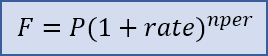Generic filters

# Compound Interest Calculator Excel Template

Excel Template Categories

Compound Interest refers to earning or paying multiple interest on interest. Although it can apply to both savings and loans, it is easiest to understand when you are thinking about savings. After each compound period, the interest earned over the period is added to the principal so the next calculation of interest includes the original principal plus the previously earned interest.

This page includes a basic compound interest calculator that you can use for simple future value problems. You can download the compound interest calculator spreadsheet to understand how compounding works from month to month.

## Compound Interest

This spreadsheet is designed as an educational tool – to help you show how compound interest works for both savings and loans. The table is based on the payment frequency and shows the amount of interest adds in each period. The graph compares the total principal and payments to the balance over time.

## Compound Interest FormulaThe basic compound interest formula for calculating a future value is F = P*(1+rate)^nper where

• F = future accumulated value
• P = principal (starting) amount
• rate  interest rate per compounding period
• nper =total number of compounding periods

### Formula for Compounding Yearly, Monthly, Weekly• P = the principal amount
• r = the nominal annual interest rate in decimal form.
• n = the number of compound periods per year
• t = the time in years

### Compound Interest formula Calculator

This is the basic formula where rate = r/n and nper = n*t. Although the math can handle a decimal value for nper, it should usually be a whole number. Traditional amortize loans use the same formulas as define for savings, except that the loan amount represents as a negative value for the starting principal, P. Payment amounts (A) are the positive values. In Excel we can use the FV function again. The formulas show how the FV function relates to the standard formula. Note that for now we aren’t including a principal amount.